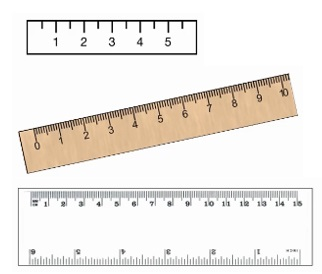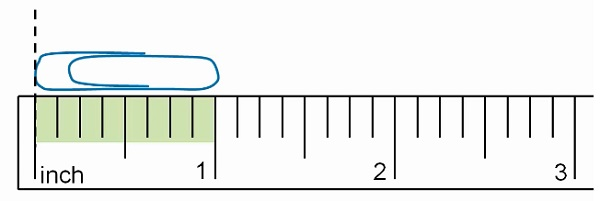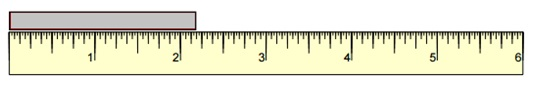# Measuring Length to the Nearest Inch

To measure distance or length we use rulers as shown.An inch is same size on all measuring tools. These rulers are marked in the US customary units of length, inches.

Taking a closer look at a ruler. There are marks on the ruler that are labeled with numbers and there are several marks between the labeled ones. We can measure the lengths to the nearest inch if we look at the labeled marks. If we want to use the lines in between, we measure length to the nearest half or quarter inch.

Two half inches go together to make an inch.

The paper clip here is exactly one-inch longMeasure the length of the paper clip to the nearest inch.### Solution

Step 1:

This paper clip is slightly more than one-inch long

We see that this paper clip is closer to the one-inch mark than to the two-inch mark.

Step 2:

So, the length of the paper clip to the nearest inch is one inch only.

Measure the length of the object to the nearest inch.### Solution

Step 1:

The reading is nearer to 2 mark than to 3 mark.

Step 2:

So, the length of the object to nearest inch is 2 inches.